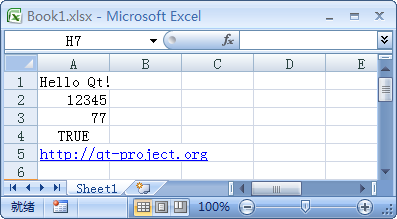# Hello QtXlsx Example

This is a simplest Qt Xlsx example.

This example demonstrates how to create a new .xlsx file containing some basic data and calculations with Qt Xlsx Library. So lets see how this is achieved.This creates a new instance of the all important Document class which gives you access to the Excel workbook and worksheets.

`QXlsx::Document xlsx;`

A default worksheet have been created by Document. Let's start by adding some basic data.

```xlsx.write("A1", "Hello Qt!");
xlsx.write("A2", 12345);
xlsx.write("A3", "=44+33");
xlsx.write("A4", true);
xlsx.write("A5", "http://qt-project.org");
xlsx.write("A6", QDate(2013, 12, 27));
xlsx.write("A7", QTime(6, 30));```

Now save the file and all its components.

`xlsx.save();`

Files: